Symmetry Properties of Orientation Functions edit page

Every SO3Fun has a left and a right symmetry. For further information on symmetries look at crystal symmetries, specimen symmetries and, Quasi symmetries.

The function values of SO3F are equal at symmetric nodes. Since the composition of rotations is not commutative there exists a left and right symmetry.

The symmetries have, for example, an influence on the plot domain.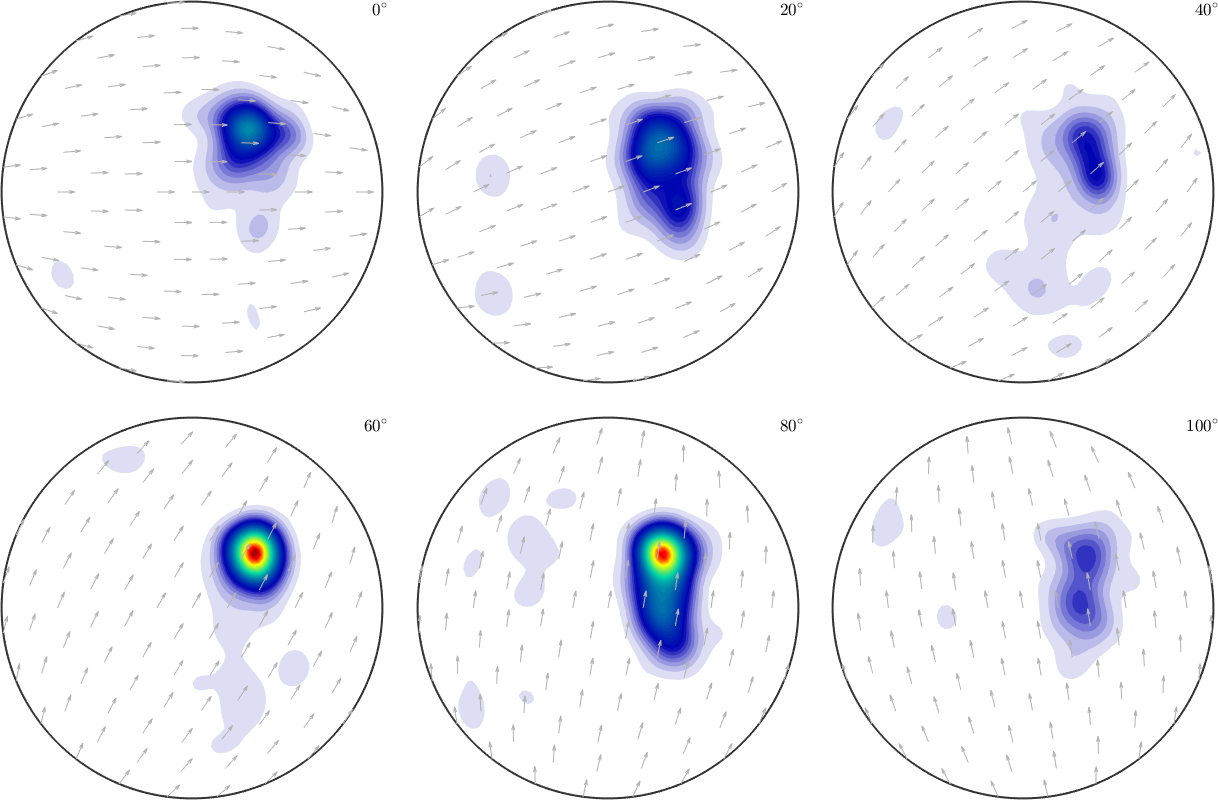• Note that only the important part with respect to the symmetry is plotted
• you can plot the full rotation group using the argument 'complete'

In most subclasses of SO3Fun the symmetries are independent from the rest of variables of the function. So one can change them very easy and only effects the function values.

The class SO3FunHarmonic describes an rotational function by the Fourier coefficients of its harmonic series. Here it is possible to get the symmetries directly from the fourier coefficients.

Similary if we want to change the symmetry of a function it is not enough to change it. We also have to symmetries this function.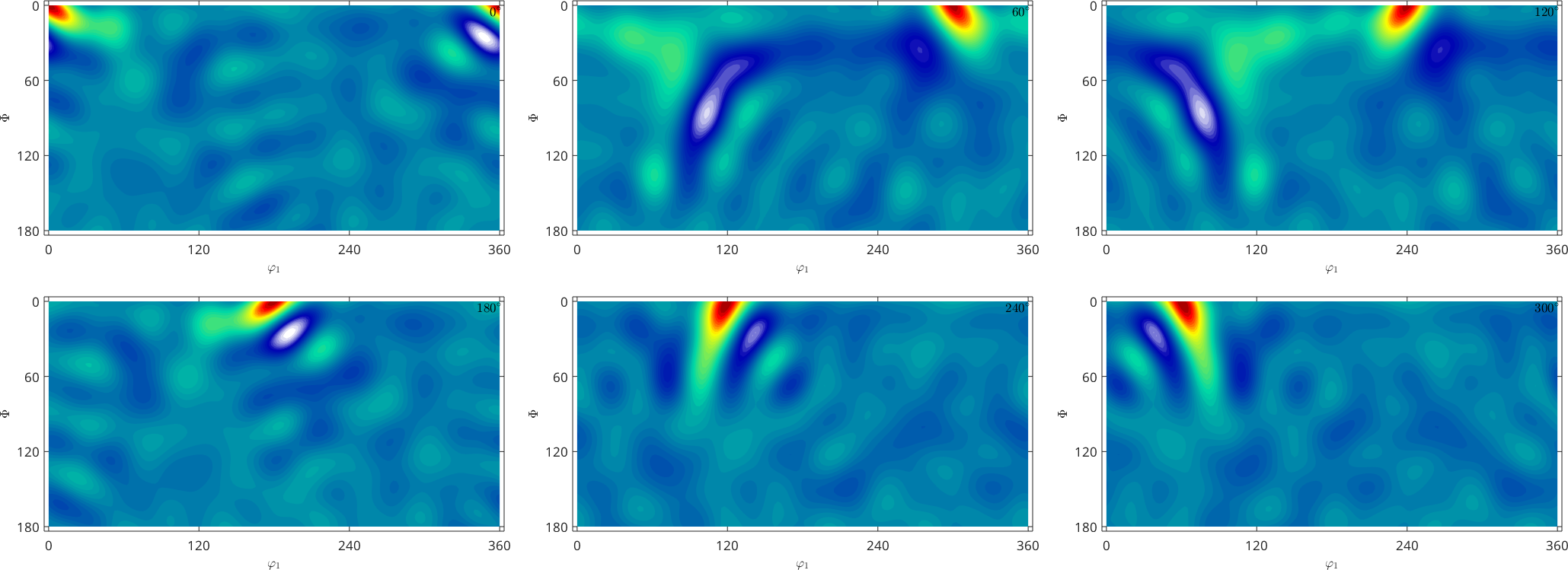Changing the symmetry has no effect on the Fourier coefficients. No we are only plotting the given function on some fundamental region.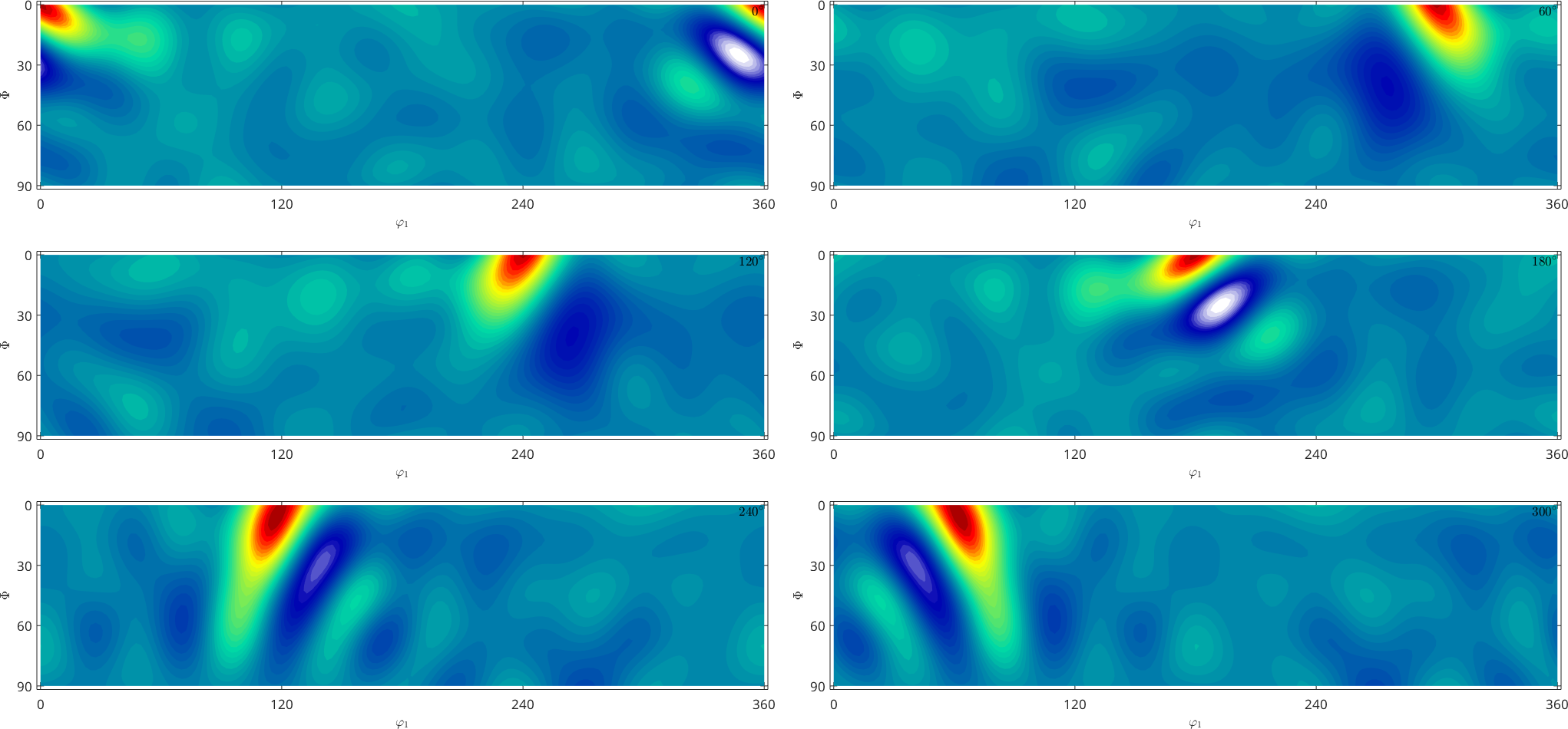Symmetrizing the Fourier coefficients transforms the coefficients. So we symmetrize the function and it is no longer possible to go back to the non symmetrized function from before.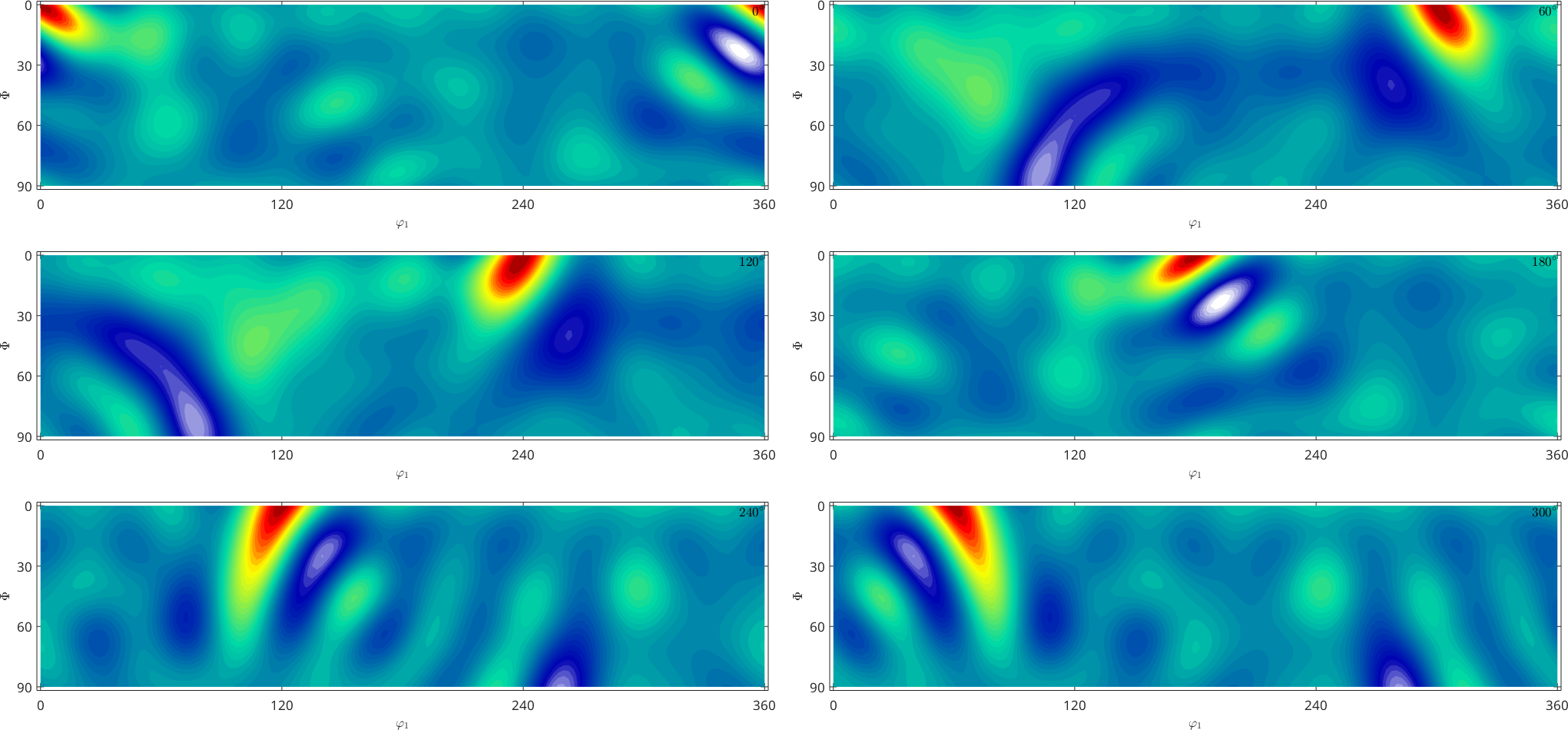Now the function is symmetrised on the full rotation group.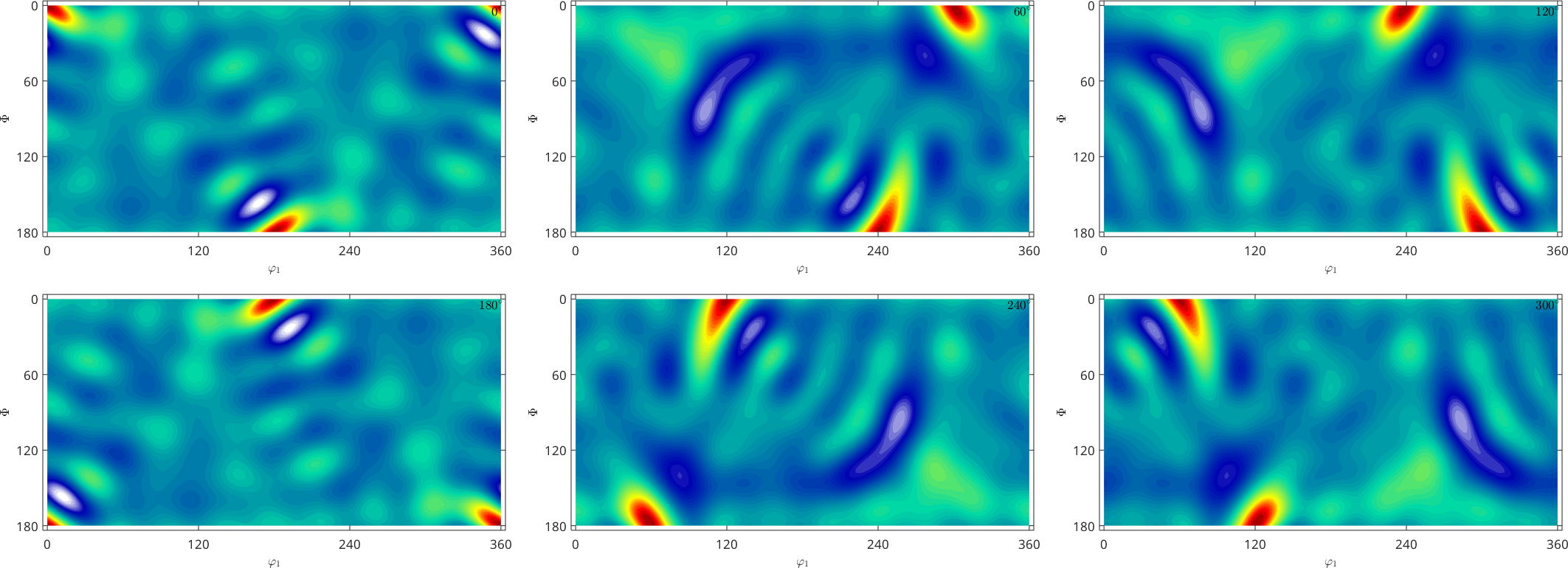Note that you can expand every SO3Fun to an SO3FunHarmonic

and do the same as before.